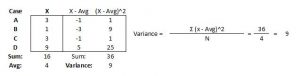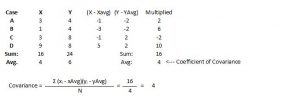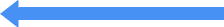# What is the Difference Between Correlation and Covariance?#### correlationarnold on Sep 17, 2008

### Correlation:

When you say that two items correlate, you are saying that the change in one item effects a change in another item. You will always talk about correlation as a range between -1 and 1. For example, if you say that two items have a correlation of .9 you are saying that a change in one item results in a similar change to another item. All the stock market indexes tend to move together in similar directions. When the DOW Jones loses 5%, the S&P 500 usually loses around 5%. When the DOW Jones gains 5%, the S&P 500 usually gains around 5% because they are highly correlated. There could also be negative correlation where you might observe that as the DOW Jones loses 5% of it value, Gold might gain 5%. Alternatively, if the Dow Jones gains 5% of its value, Gold may lose 5% of its value.

### Covariance:

If you say that two items tend to vary together then you are talking about the covariance between the two items which can be positive or negative covariance. Positive covariance indicates that higher than average values of one variable tend to be paired with higher than average values of the other variable. Negative covariance indicates that higher than average values of one variable tend to be paired with lower than average values of the other variable.

However, the number that represents covariance depends on the units of the data, so it is difficult to compare covariances among data sets that have different scales. A value that might represent a strong linear relationship for one data set might represent a very weak one in another. The correlation coefficient addresses this issue by normalizing the covariance to the product of the standard deviations of the variables, creating a dimensionless quantity that facilitates the

comparison of different data sets.

To illustrate this lets start from simple variance of one item and work our way up to correlation.When we are calculating the variance for the four numbers we are interested simply in how much the numbers vary from the mean. If all four values of X were 4, and the average was 4, then there would be no variance. If one number was 13, and the others were all 1’s, then the variance from the mean would be quite high (27). In our example the variance of the four numbers is 9.

Now lets calculate the covariance of two sets of numbers:When we calculate the covariance for the two sets of numbers we are trying to see how X and Y covary in relation to one another. The example above illustrates a basic way of calculating covariance. Lets talk about what would happen to the covariance if I changed some of the numbers. If all the values of my X are changed to 4, the average is still going to be 4, but my covariance coefficient would equal 0. If I change my X so that I have 4, 4, 5, 6, then my average will be 4, but my covariance will increase to 1.5. If my X values are arranged as 1, 1, 1, and 13, then my covariance coefficient will be 6. Conversely, if I arrange my X values to be 13,1,1 and 1, then my covariance coefficient will be -6. The main thing that causes this difference is that in the first part of the example, the 9 (13 – 4) gets multiplied by 2, and in the second example it gets multiplied by -2.

There are multiple ways to calculate covariance and I will demonstrate one more basic way in case that is how it has been presented to you in the past.The next step is to calculate the correlation. The correlation is simply calculated as:

Covariance of X and Y
———————-
SD of X * SD of Y

In our example above the covariance of X and Y is 4. The standard deviation of x is 3 and the standard deviation of Y is 2. If we use these numbers we can find the correlation of X and Y.

4
—– = .67
2 * 3

## DIGITAL MARKETING Email List

##### SPEND ONE HOUR A WEEK ON YOUR DIGITAL MARKETING WITH THE HELP OF OUR WEEKLY GUIDES EMAILED TO YOU!